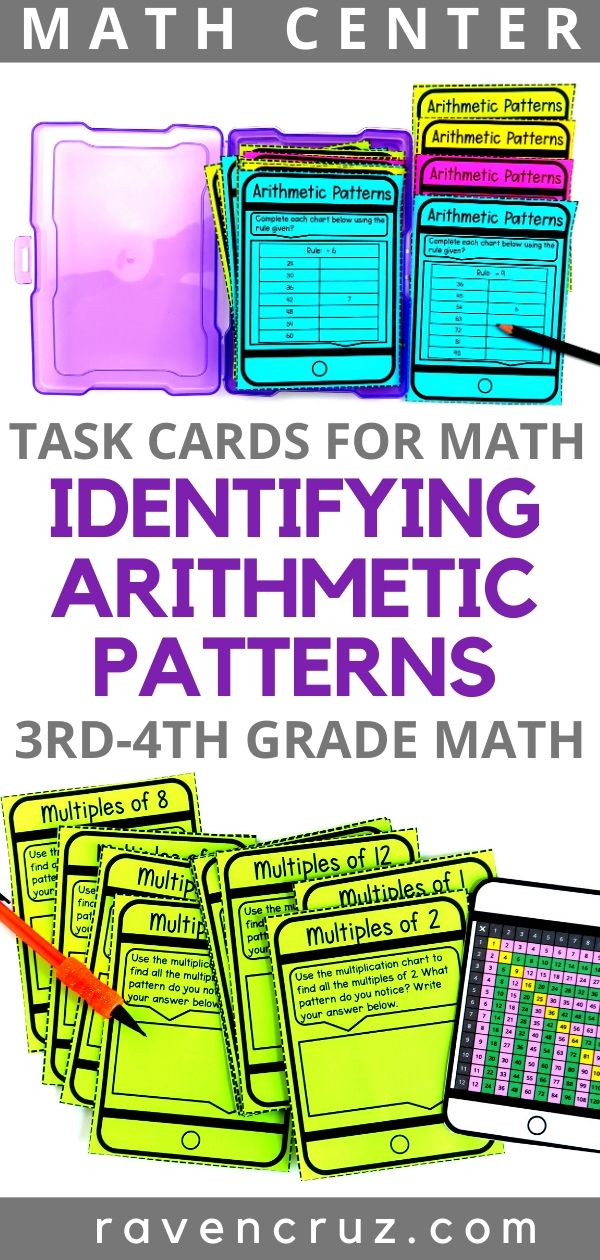Identifying Arithmetic Patterns in 3rd-Grade - Raven Cruz

# Identifying Arithmetic Patterns in 3rd-Grade

Identifying arithmetic patterns in 3rd-grade using the four operations does not have to be a complicated concept for students.

I have created two different activities to help students creatively identify arithmetic patterns. The first activity is task cards using the four operations table and the second is using a multiplication chart to identify multiples and patterns.

Controversially,  I am not going to lie, task cards are overdone. As a math teacher, I see so many task cards that "kinda" get the job done, and some that probably don't.

Alternately, there are some AMAZING standards-based task cards that obviously took much creativity and thought.

With both of these factors in mind, I created two sets of task cards that I truly feel add value and simplicity to identifying arithmetic pattern math centers for 3rd-grade math.## Arithmetic Patterns Using the Four Operations

The set of task cards shown below teach students how to find arithmetic patterns using the four operations by using a given rule. These task cards work well in math centers and math rotations because students can complete and check them independently.

I feel like these task cards step out of the 12, 15, ____, 21, 24... "find the missing number in the pattern". However, this objective has it's place, I just want to give teachers something their curriculum doesn't provide.

Click the photograph to visit the resource and decide if it's right for your class.

## Arithmetic Patterns Using Multiplication Charts

Next, task cards Identifying arithmetic patterns using multiplication charts. These task cards ask students to use the multiplication chart to find the multiples of each number. Then, ask the student to list any patterns that they notice.

Students are able to practice their problem solving skills as well as there number sense.

Like the task cards above, these work well in math centers and rotations. Teachers can write out an answer card with possible answers and students can check their work if needed.

Also, the multiplication charts are multi-purpose. You can use them for SO MANY other things in your classroom; math toolboxes, math centers, intervention, activities, etc...

Click the photograph to visit the resource and decide if it's right for your class.